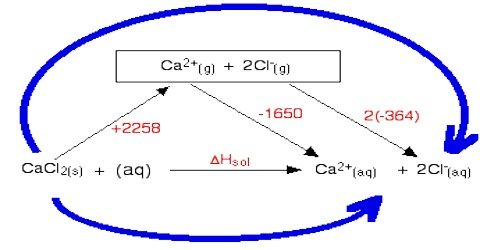# Enthalpy of Dilution

Enthalpy of Dilution

When one mole of a substance is dissolved in a specified quantity of solvent in a large number of steps the enthalpy change per mole of solid for each step is called the differential heat of solution. Sometimes it is desirable to define another enthalpy term known as enthalpy of dilution. The heat of dilution is the difference between two integral heats of solutions.

Considering example: If some ammonium chloride or glucose is added to water in a test tube the tube becomes cooler. On the other hand if some solid NaOH is added to the test tube the water becomes quite warm. These are examples of a common experience that when solids are dissolved in water heat is either absorbed or evolved.

Na+ OH (s) + H2O (l) → Na+ (aq) + OH (aq);   ∆H = – 40 kJ mol-1

NH4+ NO3 (s) + H2O (l) → NH4+ (aq) + NO3 (aq);   ∆H = + 26 kJ mol-1

The heat of a solution of NaOH in water, the heat of solution of 1.0 mole of NaOH in 5 moles of water and in 200 moles of water are respectively – 37.8 and -42.3 kJ. Thus, in the above example of enthalpy of solution of NaOH the difference between the two values, – 4.5 kJ would be the enthalpy of dilution.

On many occasions it is essential to know the enthalpy of solution at infinite dilution. If we keep on diluting a solution by gradual addition of the solvent there will be heat change at each dilution. Finally a stage will come when any further dilution produces no thermal change. This stage is called the state of infinite dilution. The enthalpy of solution at infinite dilution is defined as:

“The enthalpy change when one mole of a substance is dissolved in such a large volume of solvent so that any further dilution produces no thermal effect”.

The integral heat of solution reaches a limiting value at infinite dilution. Thus if 1.0 mole of HC1 is dissolved in a large volume of water so that no heat change is observed when more water is added one can write

HCl (g) + aq → HC1(aq);      ∆H0 = – 75.1 kJ